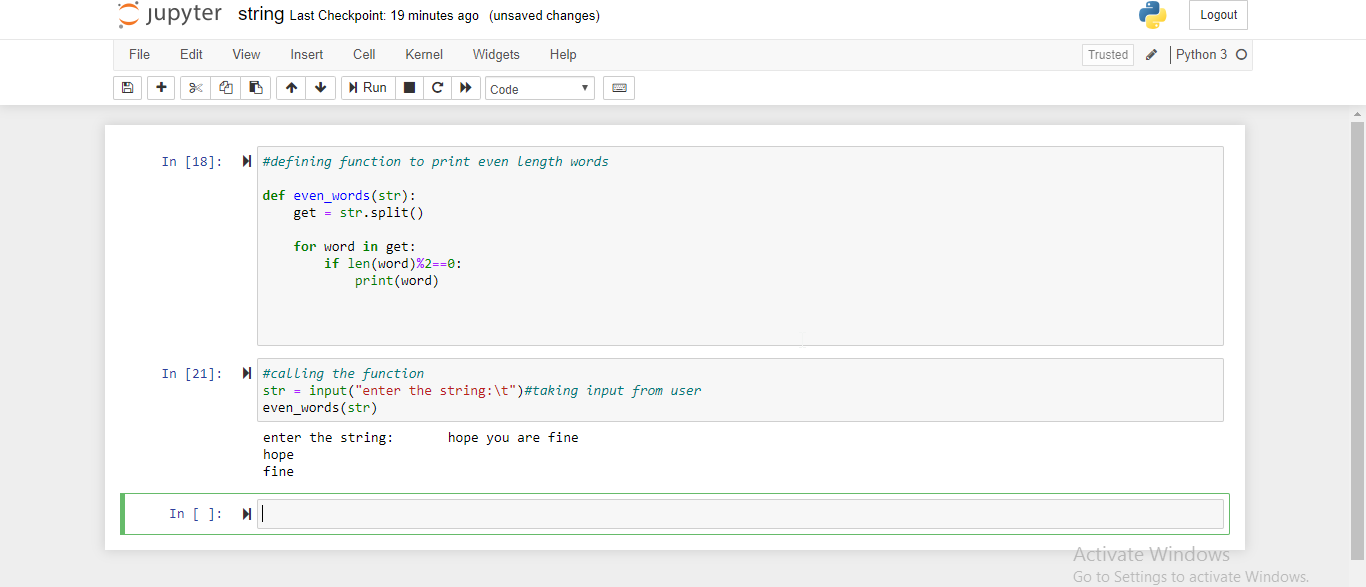# Python Program to print even length words in a String

In this article, we are gonna find the solution to the given problem statement. So, at first let’s look at the problem statement. The task that we have to do is to create a Python program that prints only even length words in a string.

Let me explain through an example:

INPUT:   str = “It was nice meeting you”

OUTPUT:

It

nice

So, if I’m entering a string as given in the above example then output are those words who have even length as shown above.

## Print only even length words in a String in Python

This is the required python program to print even length words in a string:

```#defining function to print even length words

def even_words(str):
get = str.split()

for word in get:
if len(word)%2==0:
print(word)

#calling the function
str = input("enter the string:\t")
even_words(str)```
• As you can see, we have defined a function named even_words having argument ‘str’.
• Then we have used split( ) method which will split the string that we have entered into a list and then we used for loop.
• In for loop, we checked for every word in the string that if the length of the word(by using len( ) ) is even and then we print that word.
• At last, we took input from the user and then we called the function.

#### Output:That’s it, hope this helped you.